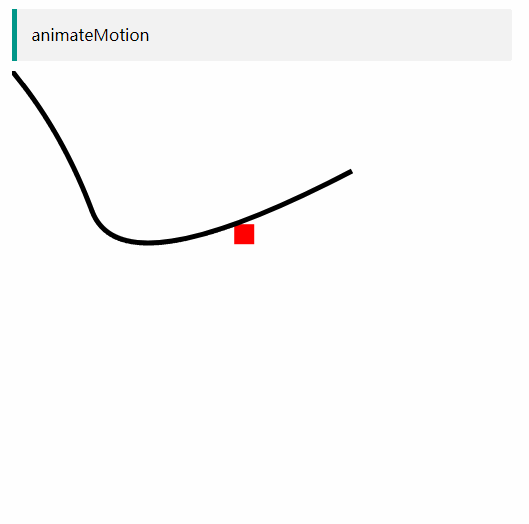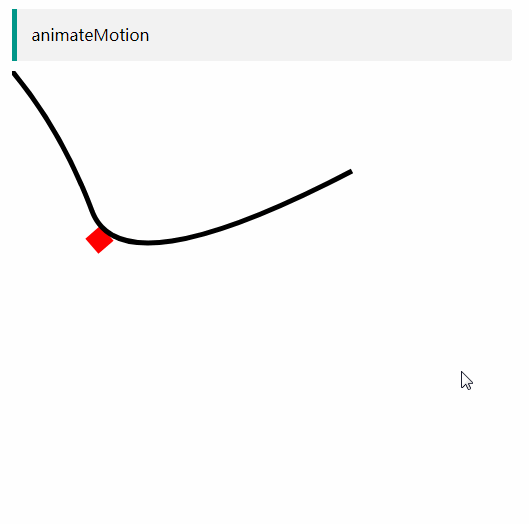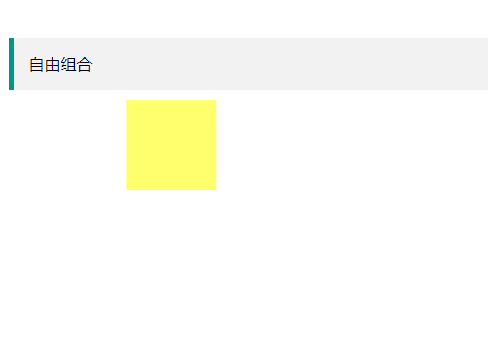# 置顶SVG动画教程精选热门

SVG动画能够实现和CSS3动画类似的效果，除此之外还能够实现强大的路径动画，也就是使物体按照一定的路径移动。让我们跟着这篇SVG动画的入门教程开始学习吧。

SVG动画能够实现和CSS3动画类似的效果，除此之外还能够实现强大的路径动画，也就是使物体按照一定的路径移动。

### SVG 动画元素介绍

svg可以设置动画的元素有5个，分别为
<set>
<animate>
<animateColor>
<animateTransform>
<animateMotion>

#### set

set用于设置属性值，可以用于实现延迟设置功能，也就是可以在特定时间之后修改某个属性值。

``````<svg height="400" width="400">
<rect width="90" height="90" fill="yellow" >
<set attributeName="x" attributeType="XML" to="60" begin="3s" />
</rect>
</svg>
``````

#### animate

``````<svg height="400" width="400">
<rect width="90" height="90" fill="yellow" >
<animate attributeName="x" from="160" to="60" begin="0s" dur="3s"/>
</rect>
</svg>
``````

#### animateTransform

``````<svg width="320" height="320" xmlns="http://www.w3.org/2000/svg">
<rect width="90" height="90" fill="yellow" >
<animateTransform attributeName="transform" begin="0s" dur="3s"  type="rotate" from="1" to="90" repeatCount="indefinite"/>
</rect>
</svg>
``````

#### animateMotion

animateMotion 元素可以用于定义SVG路径动画。如下例子(例子中的path与动画无关，只是用于标示)

``````<svg width="360" height="200" xmlns="http://www.w3.org/2000/svg">
<rect width="20" height="20" fill="red"  >
<animateMotion path="M0,0 Q50,60 80,140 T340,100" begin="0s" dur="20s" repeatCount="indefinite"/>
</rect>

<path xmlns="http://www.w3.org/2000/svg" d="M0,0 Q50,60 80,140 T340,100" id="svg_13" stroke-linecap="null" stroke-linejoin="null" stroke-dasharray="null" stroke-width="5" stroke="#000000" fill="none"/>
</svg>
````````````<svg width="360" height="200" xmlns="http://www.w3.org/2000/svg">
<rect width="20" height="20" fill="red"  >
<animateMotion path="M0,0 Q50,60 80,140 T340,100" begin="0s" dur="20s" rotate="auto" repeatCount="indefinite"/>
</rect>

<path xmlns="http://www.w3.org/2000/svg" d="M0,0 Q50,60 80,140 T340,100" id="svg_13" stroke-linecap="null" stroke-linejoin="null" stroke-dasharray="null" stroke-width="5" stroke="#000000" fill="none"/>
</svg>
``````#### 自由组合

``````<svg width="320" height="320" xmlns="http://www.w3.org/2000/svg">
<rect width="90" height="90" fill="yellow" >
<animate attributeName="x" from="160" to="60" begin="0s" dur="3s" repeatCount="indefinite" />
<animate attributeName="opacity" from="1" to="0" begin="0s" dur="3s" repeatCount="indefinite" />
</rect>
</svg>
``````### SVG 动画标签参数详解

#### 2、attributeType=“CSS | XML | auto”

attributeType支持三个固定参数"CSS | XML | auto"，用来表明定义在attributeName上面的属性值。比如我们定义的属性是属于SVG标签上的属性，那么直接设置attributeType=‘xml’，如果是设置css属性，则设置type值为css。auto为默认值，自动判别attributeName的属性是属于XML还是CSS（实际上是先当成CSS处理，如果发现不认识，直接XML类别处理）。因此，如果你不确信某属性是XML类别还是CSS类别的时候，我的建议是不设置attributeType值，直接让浏览器自己去判断，几乎无差错。

#### 3、from，to，by，values

from = “value” 动画的起始值。

to = “value” 指定动画的结束值。

by = “value” 动画的相对变化值。

values = “list” 用分号分隔的一个或多个值，可以看出是动画的多个关键值点。

from, to, by, values相互之间存在有一些制约关系。需要满足以下一些规则：

• 如果动画的起始值与元素的默认值是一样的，from参数可以省略。
• to,by两个参数至少需要有一个出现（不考虑values）。否则动画效果没有。to表示绝对值，by表示相对值。
• 如果to,by同时出现，则by打酱油，只识别to。
• values可以设置多个动画节点，不同于to/by只能设置单个动画节点，当values值有效设置了之后，from, to, by 的值都会被忽略。
``````<svg width="320" height="200" xmlns="http://www.w3.org/2000/svg">
<text font-family="microsoft yahei" font-size="120" y="150" x="160">马
<animate attributeName="x" values="160;40;160" dur="3s" repeatCount="indefinite" />
</text>
</svg>
``````

#### 4. begin, end

begin是指动画开始的时间。跟CSS3中的-delta有点像，但begin具备的功能比-delta多很多。

begin可以设置的值：

``````1、具体时间值 'h' | 'min' | 's' | 'ms'这些，默认单位是's'

2、偏移值，'+/-'，应该指相对于，某个操作的值，如begin="x.end-1s"，

3、基于另一个动画的值，如某一个动画执行完成后开始执行，如begin="x.end"

4、与事件关联的值，如点击时开始执行动画begin="circle.click"

5、与某个动画执行次数相关的，比如某个元素执行2次后开始执行begin="x.repeat(2)"

6、与键盘事件相关的，比如按下某个键开始执行 begin="accessKey(s)"
``````
``````<svg width="320" height="200" xmlns="http://www.w3.org/2000/svg">
<text font-family="microsoft yahei" font-size="120" y="160" x="160">马
<animate attributeName="x" to="60" begin="accessKey(s)" dur="3s" repeatCount="indefinite" />
</text>
</svg>
``````

end 个属性跟begin相对，属性值的有效类型一样。

#### 5. dur

‘indefinite’。设置’indefinite’跟没有设置动画是一个意思。

#### 6. calcMode, keyTimes, keySplines

1、calcMode

• discrete：不连续的运动，就是从一个状态直接跳到另一个状态下，如从from状态直接跳到to状态
• linear：线性运动，animateMotion元素以外元素的calcMode默认值。动画从头到尾的速率都是一致的。每个动画阶段的执行时间都是一致。
• paced ：线性运动，动画从头到尾执行速度都是一样，如果设置了paced，keyTimes无效。
• spline ： 属于可以自定义执行速度，类似于-timing-function: cubic-bezier()这一CSS属性。我们可以通过keyTimes和keySplines属性来定义各个动画的执行效果。
``````<svg version="1.1" xmlns="http://www.w3.org/2000/svg">
<rect x="10" y="10" width="50" height="50" fill="blue">
<animate xlink:href="#ant" attributeName="x" calcMode="discrete" values="10;30;150" dur="4s"/>
</rect>

<rect x="10" y="60" width="50" height="50" fill="blue">
<animate xlink:href="#ant" attributeName="x" calcMode="linear" values="10;30;150" dur="4s" />
</rect>

<rect x="10" y="110" width="50" height="50" fill="blue">
<animate xlink:href="#ant" attributeName="x" calcMode="paced" values="10;30;150" dur="4s"/>
</rect>

<rect x="10" y="160" width="50" height="50" fill="blue">
<animate xlink:href="#ant" attributeName="x" calcMode="spline" values="10;30;150" keyTimes="0; .8; 1" dur="4s" />
</rect>
</svg>
``````

2、keyTimes

keyTimes用于限定各个阶段的执行时间，是一串0-1的浮点数字组，每个数值的有效取值范围为0-1，代表每一个动画阶段必须在那个时间点执行完成。

keyTimes的数字组长度要和values长度一直，如果是to/by动画就只有两个值。否则会当做无效处理。

``````<svg version="1.1" xmlns="http://www.w3.org/2000/svg">

<rect x="10" y="10" width="50" height="50" fill="blue">
<animate xlink:href="#ant" attributeName="x" calcMode="spline" values="10;90;150" keyTimes="0; .8; 1" dur="4s"/>
</rect>

<rect x="10" y="60" width="50" height="50" fill="blue">
<animate xlink:href="#ant" attributeName="x" calcMode="spline" values="10;90;150" keyTimes="0; .2; 1" dur="4s"/>
</rect>
</svg>
``````

3、keySplines

``````<svg version="1.1" xmlns="http://www.w3.org/2000/svg">
<rect x="10" y="10" width="50" height="50" fill="blue">
<animate xlink:href="#ant" attributeName="x" calcMode="spline" values="10;90;150" keyTimes="0; .8; 1" keySplines="0.42 0 1 1;0 0 0.59 1;0.42 0 1 1;" dur="4s"/>
</rect>
</svg>
``````

#### 7. repeatCount, repeatDur

repeatCount表示动画执行次数，可以是合法数值或者"indefinite"。

repeatDur定义重复动画的总时间。可以是普通时间值或者"indefinite"。

#### 8. fill

fill表示动画间隙的填充方式。支持参数有：freeze | remove。

• remove ：是默认值，表示动画结束直接回到开始的地方。
• freeze ：表示动画结束后元素固定在结束的位置。

accumulate是累积的意思。支持参数有：none | sum. 默认值是none。如果值是sum表示动画结束时候的位置作为下次动画的起始位置。

``````<svg version="1.1" xmlns="http://www.w3.org/2000/svg">
<rect x="10" y="10" width="50" height="50" fill="blue">
<animateTransform attributeName="transform" type="scale" from="1" to="3" dur="10s" repeatCount="indefinite" additive="sum"/>
<animateTransform attributeName="transform" type="rotate" from="0 30 20" to="360 30 20" dur="10s" fill="freeze" repeatCount="indefinite" additive="sum"/>;
</rect>
</svg>

``````

#### 10. restart

• always是默认值，表示总是，也就是没触发一次动画执行一次。
• whenNotActive：表示动画正在进行的时候，是不能重启动画的。
• never：表示动画不能被重新触发。

### 动画暂停裕播放

SVG 提供一些js接口可以用于控制动画

// 暂停
svg.pauseAnimations();

// 重启动
svg.unpauseAnimations();

### 参考文章